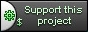# Difference "Serialization" ver. 3 versus ver. 4

## Content:

 `` `## Introduction` `It is frequently required to save some C++ objects in some type of storage like user session or cache and retrieve` `them back.` `It is not a trivial task for C++ - non-reflective language. So CppCMS provide simple serialization library that helps` `us to complete such tasks.` `## CppCMS Serialization Tools` `### Preparing Objects` `Lets assume we have a class `person` that holds some information and a list of his children - `child` classes.` `Let's assume we have a class `person` that holds some information and a list of his children - `child` classes.` `Person:` ` struct person {` ` std::string name;` ` std::string family;` ` std::string occupation;` ` std::string martial_state;` ` double salary;` ` physical parameters;` ` std::list children;` ` std::vector friends;` ` };` `Child:` ` struct child {` ` std::string name;` ` physical parameters;` ` }` `Physical data about them:` ` struct physical {` ` int age;` ` double height;` ` };` `Now, in order to serialize our object we would derive` `them from `cppcms::serializable` class.` `Include `` header, and update` `the object such that:` ` struct child {` ` ...` ` };` ` struct person {` ` ...` ` };` `Become` ` struct child : public cppcms::serializable {` ` ...` ` };` ` struct person : public cppcms::serializable {` ` ...` ` };` `Note, because "physical" object is POD we would handle` `it in a better way.` `Now we add a new member function to the two` `classes above that describes how to serialize them:` `Child:` ` void serialize(cppcms::archive &a)` ` {` ` a & name & cppcms::as_pod(parameters);` ` }` `It receives as parameter `cppcms::archive` - an object` `that is capable to save/restore that data` `and we mark each one of the members: `name` ` `and `parameters`.` `Note, because we know that `parameters` is POD object` `we use special marker that describes how to copy it.` `Next we do the same for person:` ` void serialize(cppcms::archive &a)` ` {` ` a & name & family & occupation & martial_state` ` & salary & cppcms::as_pod(parameters) & children & friends;` ` }` `**Note:**` `The `archive` object knows how to deal with standard C++ containers like `std::vector` or `std::string`. It knows` `how to save primitive types like `double` as plain binary data.` `So for example because `children` is `std::list`` `and `child` is derived from `cppcms::serializable` it ` `knows how to handle this situation automatically.` `If you use your own containers or non-standard containers` `you may want to specialize: [`cppcms::archive_traits`](/cppcms_ref_v0_99/structcppcms_1_1archive__traits.html)` `class for your specific class.` `### Saving and Restoring Objects` `Let's assume that `std::string buffer` us our data container` `Let's assume that `std::string buffer` is our data container` `So we can save the data to the buffer using these` `steps:` ` person john;` ` ... // setup its data` ` cppcms::archive a;` ` john.save(a); // save it to archvive` ` buffer = a.str(); // get the buffer` `We can load it as following:` ` person john;` ` cppcms::archive a;` ` a.str(buffer);` ` john.load(a);` `This is very simple way to store and load data but we` `recommend to use little bit complicated API that` `would give us more power as we would see later:` `Save:` ` person john;` ` ... // setup its data` ` cppcms::serialization_traits::save(john,buffer);` `Load:` ` person john;` ` cppcms::serialization_traits::load(buffer,john);` `### Using with Cache and Sessions` `Now lets see how we can use CppCMS serialization` `Now let's see how we can use CppCMS serialization` `tools with Cache and Session interfaces:` `We can easily cache person objects like this:` ` person john;` ` if(!cache().fetch_data("john",john)) {` ` init_person(john);` ` cache().store_data("john",john);` ` }` `This would allow us for example reduce complex` `queries to database for some common data that is` `not updated` `We can store the objects withing the session:` ` person me;` ` if(session().is_set("me")) {` ` session().fetch_data("me",me);` ` me.salary += 1;` ` session().store_data("me",me);` ` }` `## Using 3rd Party Serialization API` `### Approach` `CppCMS API is simple fast and powerful, but it is strictly` `platform dependent - it does not allow to pass same` `object between hosts with different architecture ` `or endianess, it does not support versioning etc.` `So sometimes you want to use some 3rd party API with` `CppCMS. ` `It is quite simple, all you need to do is to provide` `specialization for `serialization_traits`` `class - that was the reason we recommended to use` ``serialization_traits` class rather then `cppcms::archive`` `directly.` `### Boost.Serialization` `Lets show how we use for example Boost.Serialization` `Let's show how we use for example Boost.Serialization` `with CppCMS.` `This is our very simple class that supports Boost.Serialization:` ` struct person {` ` std::string name;` ` std::string family;` ` template` ` void serialize(Archive &a,unsigned /*version*/)` ` {` ` a & name & family;` ` }` ` };` `Let's define two small helper functions to save` `and load the object to the string:` ` template` ` void load_from_boost(std::string const &src,T &obj)` ` {` ` std::stringbuf ss(src);` ` {` ` boost::archive::binary_iarchive oa(ss);` ` oa >> obj;` ` }` ` }` ` template` ` void save_with_boost(T const &obj,std::string &tgt)` ` {` ` std::stringbuf ss;` ` {` ` boost::archive::binary_oarchive oa(ss);` ` oa << obj;` ` }` ` tgt=ss.str();` ` }` `Now all we need is to specialize our `serialization_traits`` `to handle `person` properly:` ` namespace cppcms {` ` template<>` ` struct serialization_traits {` ` static void save(person const &obj,std::string &tgt)` ` {` ` save_with_boost(obj,tgt);` ` }` ` static void load(std::string const &src,person &obj)` ` {` ` load_from_boost(src,obj);` ` }` ` };` ` }` `Then you can freely save and load object using CppCMS` `session or cache interface:` ` person john;` ` if(!cache().fetch_data("john",john)) {` ` init_person(john);` ` cache().store_data("john",john);` ` }`

CppCMS is a web development framework for performance demanding applications.

## Support This Project